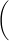# Mathnjoud

Let

f(x) = 6x2 − 4x.

(a) Use the definition of derivative and the Procedure/Example box in this section to find the derivative.
f '(x) =

(b) Find the instantaneous rate of change of f(x) at x = −1.

(c) Find the slope of the tangent to the graph of y = f(x) at x = −1.

(d) Find the point on the graph of y = f(x) at x = −1.
(x, y) =• Posted: 21 days ago
• Due:
• Budget: \$2

Purchase the answer to view itPurchase the answer to view itPurchase the answer to view itPurchase the answer to view it